Area And Perimeter - Definition with Examples

The Complete K-5 Math Learning Program Built for Your Child

• 30 Million Kids

Loved by kids and parent worldwide

• 50,000 Schools

Trusted by teachers across schools

• Comprehensive Curriculum

Aligned to Common Core

Area and Perimeter

Keywords: perimeter, area, rectangle, unit squares, square, triangle, practice, math, sides

Perimeter for a 2-dimensional shape is the total distance around the respective shape. For the figures with straight sides such as triangle, rectangle, square or a polygon; the perimeter is the sum of lengths for all the sides.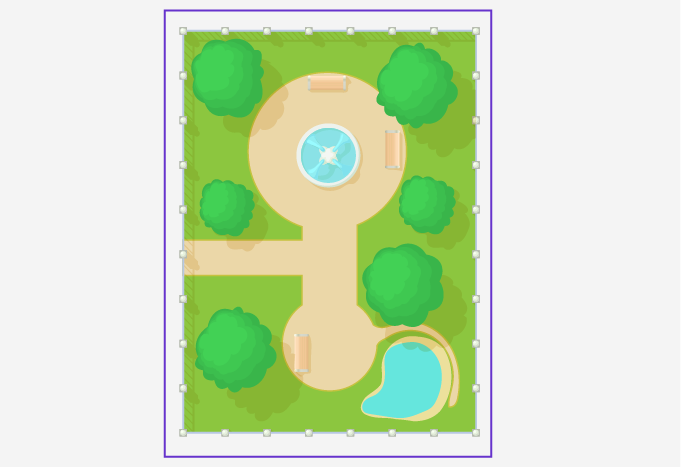The peripheral border in blue is the perimeter of the park.

Area

The area for a 2-dimensional shape is the space enclosed within the perimeter of the given shape. To calculate the area for different shapes, use different formulas based on the number of sides and other characteristics such as angles between the sides.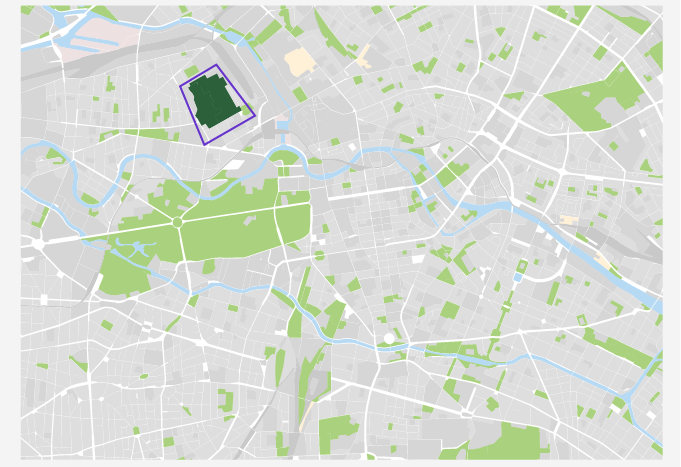The area for the park is shown in dark green color.

Calculating area and perimeter for different shapes

Triangle

The formulas for calculating the perimeter and area of a triangle ABC are: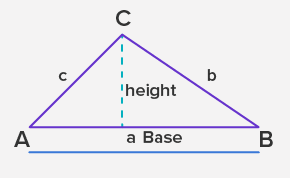• Perimeter = a + b + c

⇒ Perimeter = sum of the length of all sides

⇒ Perimeter = a + b + c

• Area = 12 × base × height

Square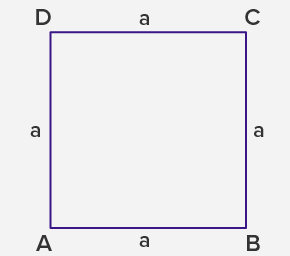• Perimeter = 4a

⇒ Perimeter = sum of lengths of all sides

⇒ Perimeter = a + a + a + a

⇒ Perimeter = 4a

• Area = a2

⇒ Area = length × breadth

⇒ area = a × a

⇒ area = a2

Rectangle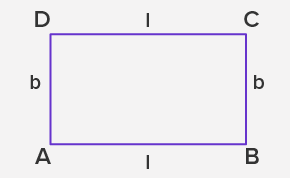• Perimeter = 2 (l + b)

⇒ Perimeter = sum of lengths of all sides

⇒ Perimeter = l + b + l + b

⇒ Perimeter = 2 × (l + b)

• Area = l × b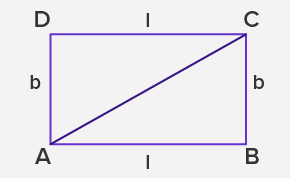⇒ Area = Area (ABC) + Area (ADC)

⇒ Area = 2 × Area (ABC)

⇒ Area = 2 × (12 × base × height)

⇒ Area = base × height

⇒ Area = l × b

Units of measurement

If all the measurements are in centimeter, the units of measurement for the perimeter and area of different shapes are:

• Perimeter = sum of sides

Thus, the unit of measurement remains the same, as cm

• Area = product of sides

The unit of measurement is unit2 or cm2

Application

The concepts of area and perimeter are the basis for understanding Euclidean geometry and calculating the volume of solid shapes in 3-dimensional space such as cones, prism, sphere, and cylinder. Also, we use these formulas for calculating the area and perimeter for quadrilaterals and polygons comprising of sides and curves. The real-life utility of the concept is in several fields such as mapping, architecture, and surveying. The geometric representation of figures is done by sketching the distances and areas for clear understanding.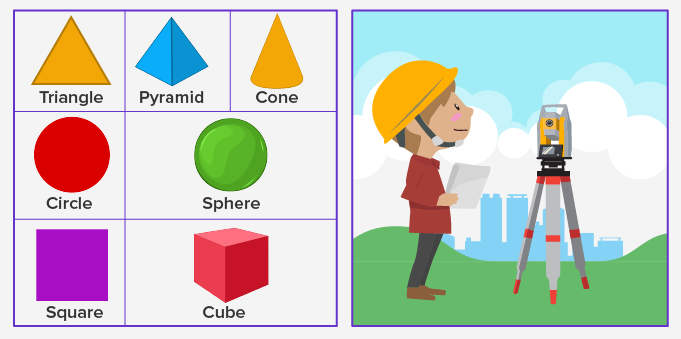Three-dimensional objects derived from 2-dimensional shapes and land surveying in fields.

 Fun Facts Different rectangles with the same perimeter can have different areas. Rectangles are also known as elongated squares. Perimeter for squares and rectangles is always less than their area, whereas it can be more than the area in case of triangles.

Won Numerous Awards & Honors